# Fractions - math word problems

1. MO Z9–I–2 - 2017In the VODY trapezoid, VO is a longer base and the diagonal intersection K divides the VD line in a 3:2 ratio. The area of the KOV triangle is 13.5 cm2. Find the area of the entire trapezoid.
2. Excavation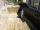Excavation for the base of the cottage 4.5 m x 3.24 m x 60 cm. The excavated soil will increase its volume by one-quarter. Calculate the volume of excavated soil.
3. Points on line segmentPoints P & Q belong to segment AB. If AB=a, AP = 2PQ = 2QB, find the distance: between point A and the midpoint of the segment QB.
4. Interior designer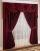To make draperies an interior designer needs 11 1/4 yards of material for the den and 8 1/2 yards for the living room. If material comes only in 20 yard bolts, how much will be left over after completing both sets of draperies?
5. The bunsKate, Zofia and Peter Liked buns. Even today, their grandmother prepare their favorite meal. Katka eats 4 bunches, Žofia 3 and Petra eats 5 buns. Their grandmother said to them, "My inmate will you know how many buns I have been make today, if those you e
6. Simple equation 324 = n • 27, solve for n
7. Chicks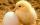How many chicks were hatched from 4500 eggs, when an average of 100 eggs hatched 87 chicks?
8. Kilo candyOne kilogram of chocolate candy cost 10 euros. Kate buys 250 grams and had the 8 pack of candy. How much does cost one bonbon?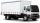How many products weighing 12.5 kg can be loaded on a cargo car with a load of 1.5 t to load two-thirds?
10. Image reduction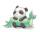To say that I am mathematically challenged is a gross understatement! I'm trying to help my friend determine how to calculate percentages of reduction or enlargement for a quilt pattern. She is creating a drawing of a cute picture which will become a pi
11. Ten fractionsWrite ten fractions between 1/3 and 2/3
12. Simple equation 2Find X in this simple equation: X/9 = 96/108
13. Passenger boatTwo-fifths of the passengers in the passenger boat were boys. 1/3 of them were girls and the rest were adult. If there were 60 passengers in the boat, how many more boys than adult were there?
14. NotebooksLiza a store owner buys 560 notebooks. He sold 3/8 of the notebook then she adds the stocks of notebook of 1/4 of the number of notebooks she bought. What is the total number of notebook she bought?
15. TeacherTeacher Rem bought 360 pieces of cupcakes for the outreach program of their school. 5/9 of the cupcakes were chocolate flavor and 1/4 wete pandan flavor and the rest were a vanilla flavor. How much more pandan flavor cupcakes than vanilla flavor?
16. Two numbersThe sum of the two numbers is 1. Find both numbers if you know that half of the first is equal to one seventh of the second number.
17. Ratio of two unknown numbersTwo numbers are given. Their sum is 30. We calculate one-sixth of a larger number and add to both numbers. So we get new numbers whose ratio is 5:7. Which two numbers were given?
18. Direct route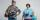From two different places A and B connected by a direct route, Adam (from city A) and Bohus (from city B) started at a constant speed. As Adam continued to go from A to B, Bohus turned around at the time of their meeting and at the same speed he returned
19. Quotient 3If the quotient of 8/13 and 2 is subtracted from the product of 1 3/4 and 8/21, what is the difference?
20. Ratio 11Simplify this ratio 10 : 1/4

Do you have an interesting mathematical word problem that you can't solve it? Submit math problem, and we can try to solve it.

We will send a solution to your e-mail address. Solved examples are also published here. Please enter the e-mail correctly and check whether you don't have a full mailbox.

Please do not submit problems from current active competitions such as Mathematical Olympiad, correspondence seminars etc...

Need help calculate sum, simplify or multiply fractions? Try our fraction calculator.